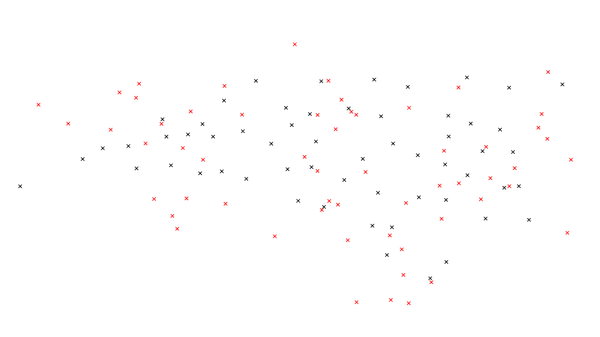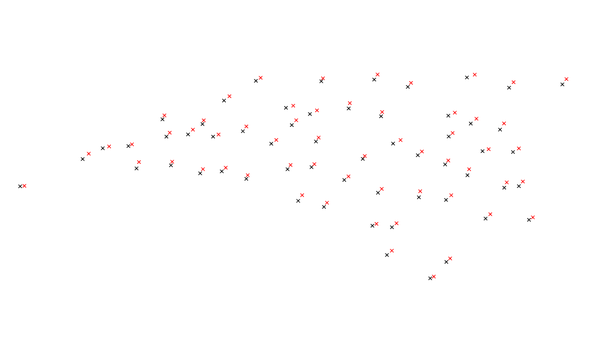Note: This document is for an older version of GRASS GIS that will be discontinued soon. You should upgrade, and read the current manual page.

## NAME

v.perturb - Random location perturbations of vector points.

## KEYWORDS

vector, geometry, statistics, random, point pattern, level1

## SYNOPSIS

v.perturb
v.perturb --help
v.perturb [-sb] input=name [layer=string] output=name [distribution=string] parameters=float[,float,...] [minimum=float] [seed=integer] [--overwrite] [--help] [--verbose] [--quiet] [--ui]

### Flags:

-s
Generate random seed (result is non-deterministic)
-b
Do not build topology
Advantageous when handling a large number of points
--overwrite
Allow output files to overwrite existing files
--help
Print usage summary
--verbose
Verbose module output
--quiet
Quiet module output
--ui
Force launching GUI dialog

### Parameters:

input=name [required]
Name of input vector map
Or data source for direct OGR access
layer=string
Layer number or name ('-1' for all layers)
A single vector map can be connected to multiple database tables. This number determines which table to use. When used with direct OGR access this is the layer name.
Default: -1
output=name [required]
Name for output vector map
distribution=string
Distribution of perturbation
Options: uniform, normal
Default: uniform
parameters=float[,float,...] [required]
Parameter(s) of distribution
If the distribution is uniform, only one parameter, the maximum, is needed. For a normal distribution, two parameters, the mean and standard deviation, are required.
minimum=float
Minimum deviation in map units
Default: 0.0
seed=integer
Seed for random number generation

## DESCRIPTION

v.perturb reads a vector map of points and writes the same points but perturbs the eastings and northings by adding either a uniform or normal delta value. Perturbation means that a variating spatial deviation is added to the coordinates.

## NOTES

The uniform distribution is always centered about zero. The associated parameter is constrained to be positive and specifies the maximum of the distribution; the minimum is the negation of that parameter. Do perturb into a ring around the center, the minimum parameter can be used.

Usually, the mean (first parameter) of the normal distribution is zero (i.e., the distribution is centered at zero). The standard deviation (second parameter) is naturally constrained to be positive.

Output vector points are not guaranteed to be contained within the current geographic region.

## EXAMPLES

### Random, uniformly distributed selection

To create a random, uniformly distributed selection of possible new points with a radius of 100,000 map units, use the following command:
```v.perturb input=comm_colleges output=uniform_perturb parameters=100000
```
Your map should look similar to this figure:Figure: Map showing the actual community college points and uniformly random chosen points.

### Normal distributed selection

For a normal distribution with a mean of 5000 and standard deviation of 2000, use the following command:
```v.perturb input=comm_colleges output=normal_perturb distribution=normal parameters=5000,2000
```Figure: Map showing the actual community college points and normally random chosen and colored points. Notice that each point is closer to the original point.

### Normal distributed selection with a minimum value

In order to include a minimum value of 500, use the following command:
```v.perturb input=comm_colleges output=min_perturb distribution=normal parameters=100000,1000 minimum=500
```

v.random, v.univar

## AUTHORS

James Darrell McCauley
when he was at: Agricultural Engineering Purdue University

Random number generators originally written in FORTRAN by Wes Peterson and translated to C using f2c.

## SOURCE CODE

Available at: v.perturb source code (history)

Latest change: Thursday Feb 03 11:10:06 2022 in commit: 547ff44e6aecfb4c9cbf6a4717fc14e521bec0be

Note: This document is for an older version of GRASS GIS that will be discontinued soon. You should upgrade, and read the current manual page.

© 2003-2023 GRASS Development Team, GRASS GIS 8.2.2dev Reference Manual